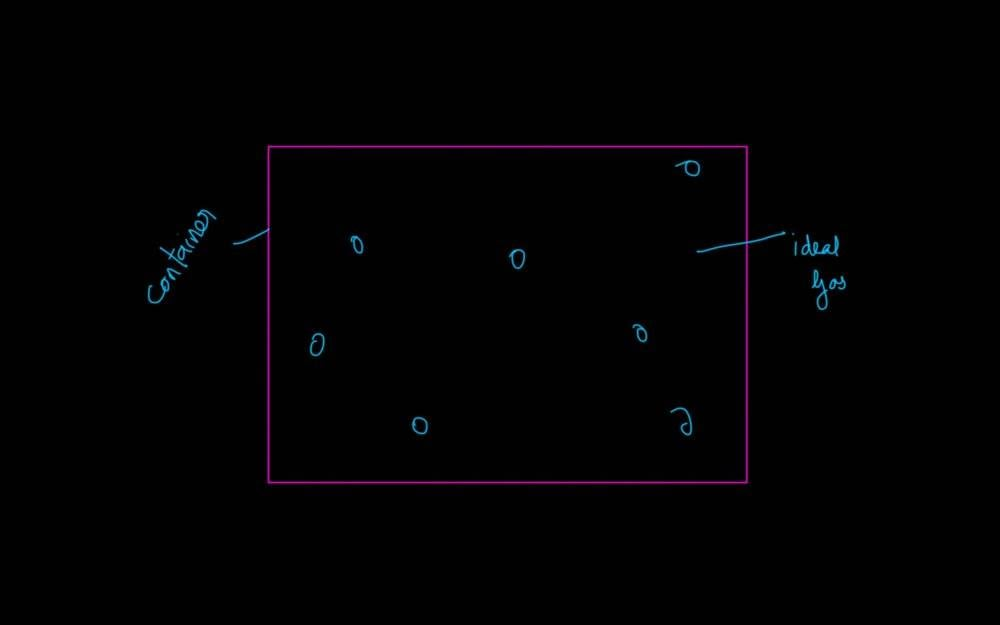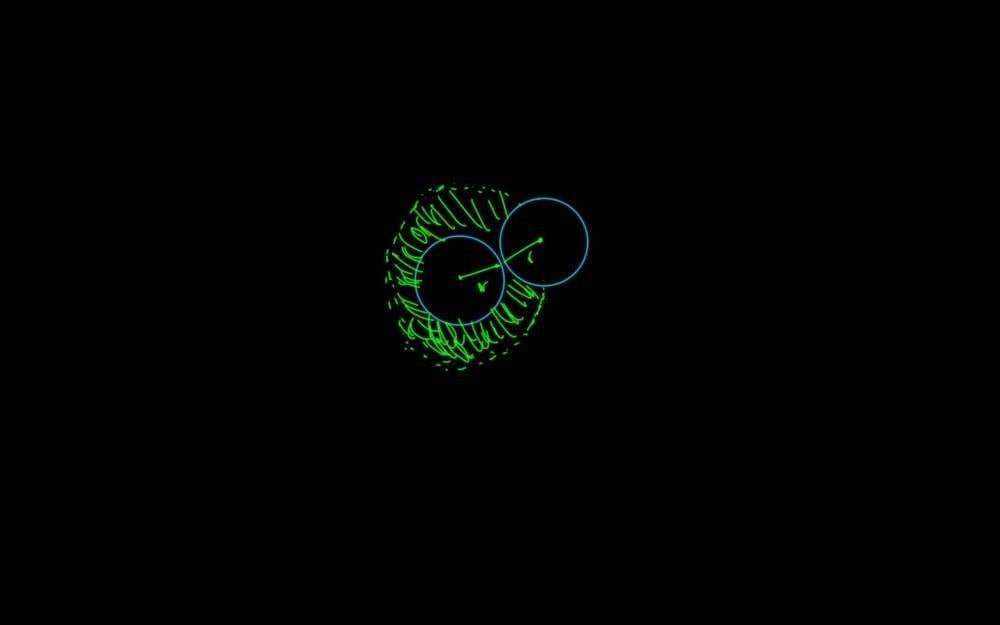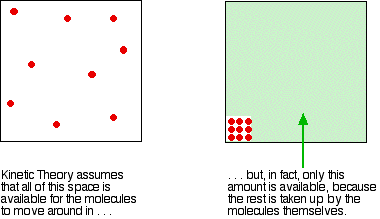# Van der Waals constant 'b' for a real gas

Kaushik
TL;DR Summary
• Doesn't volume of ideal gas include volume of the molecules?• What I was taught in school is that, when two molecules collide, no other molecule can come around it (inside the green part in the figure) and that volume is excluded volume? Is it correct? If yes, why?An ideal gas is composed of point particles with zero volume that only make elastic collisions. When you insert more realistic proerties of real gases, such as the volume taken up by each particle and the interactions between the particles, then the gas no longer obeys the ideal gas equation, but obeys the Van der Waals equation. Real gases are much better described by the Van der Waals equation than by the ideal gas equation.

•Kaushik
Kaushik
An ideal gas is composed of point particles with zero volume that only make elastic collisions. When you insert more realistic properties of real gases, such as the volume taken up by each particle and the interactions between the particles, then the gas no longer obeys the ideal gas equation, but obeys the Van der Waals equation. Real gases are much better described by the Van der Waals equation than by the ideal gas equation.
When we talk about volume of gases, is it the volume of the container, or the volume of the molecules or is it both?

Let Ideal Gas A be kept in a container of Volume V. Is ##V_{ideal} = V_{container}##?

Last edited:
Mentor
In general by "volume of a gas" we mean volume it occupies - so the volume of the container. That in turn is sum of volume of the gas molecules (let's not get into details, as they are a bit fuzzy) and the space between them.

•Kaushik
Kaushik
The kinetic theory assumes that, for an ideal gas, the volume taken up by the molecules themselves is entirely negligible compared with the volume of the container. For a real gas, that assumption isn't true. The molecules themselves do take up a proportion of the space in the container. The space in the container available for things to move around in is less than the measured volume of the container.Source

After reading the above, my interpretation of volume of gas is that the space for the molecules to move around. When we consider the volume of molecules, then the space for it to move around reduces.
But according to my interpretation the volume of real gas should be lower than the volume of ideal gas which is not true.

In general by "volume of a gas" we mean volume it occupies - so the volume of the container. That in turn is sum of volume of the gas molecules (let's not get into details, as they are a bit fuzzy) and the space between them.
But when we talk about volume of ideal gas, we are considering the volume of the container which is the sum of volume of molecules and the space in between them. Then what do we take into account when we say volume of molecule is negligible as compared to volume of container?

What I was taught in school is that, when two molecules collide, no other molecule can come around it (inside the green part in the figure) and that volume is excluded volume? Is it correct? If yes, why?
What is the reason for this? (find the figure attached above)

Last edited:
Let Ideal Gas A be kept in a container of Volume V. Is ##V_{ideal} = V_{container}##?
Yes
After reading the above, my interpretation of volume of gas is that the space for the molecules to move around. When we consider the volume of molecules, then the space for it to move around reduces.
But according to my interpretation the volume of real gas must be lower than the volume of ideal gas which is not true.
But when we talk about volume of ideal gas, we are considering the volume of the container which is the sum of volume of molecules and the space in between them. Then what do we take into account when we say volume of molecule is negligible as compared to volume of container?
I don't understand your question here. If you look at the Van der Waals equation,
$$(P+a \frac{n^2}{V^2})(V-nb) = nRT$$
the volume is in fact reduced by the factor nb because of the finite volume of the gas molecules. Why do you say, "the volume of real gas must be lower than the volume of ideal gas which is not true."

•Kaushik
Kaushik
Why do you say, "the volume of real gas must be lower than the volume of ideal gas which is not true."
I know that it is not true. But I can't understand why that is the case.

I know that it is not true. But I can't understand why that is the case.
I don't know what else to say except to repeat that it is because the molecules themselves take up some space.

•Kaushik
Kaushik
I don't know what else to say except to repeat that it is because the molecules themselves take up some space.
Can you answer the below question?
But when we talk about volume of ideal gas, we are considering the volume of the container which is the sum of volume of molecules and the space in between them. Then what do we take into account when we say volume of molecule is negligible as compared to volume of container?

In an ideal gas, the volume of the molecules is zero, so of course zero is negligible as compared to the volume of the container. Saying that something is negligibly small is another way of saying that we will assume that it is zero.

You do realize that ideal gasses do not exist, right?

•Kaushik
Kaushik
Yes
So you said that ## V_{container} = V_{ideal}## .
Then what is volume of real gas?
Is it ## V_{real} = V_{container}+V_{molecules}##?
If that is true, my question is 'Doesn't Volume of the container already include volume of the molecules as they are inside the container?'

I'm completely lost as to what your question is. I think this is an obvious concept and you are just trolling. I have done my best to try and explain, but I am dropping out of this thread now.

•Kaushik
Mentor
Apparently confusion stems from the fact "volume of the gas" is ambiguous and can mean both "volume it occupies" and "volume of the molecules themselves". As long as you don't start to pay attention to what you really mean things won't get better.

•Lord Jestocost and Kaushik
Homework Helper
What I was taught in school is that, when two molecules collide, no other molecule can come around it (inside the green part in the figure) and that volume is excluded volume? Is it correct? If yes, why?
Could it be that this is what you remember, not what you were taught ?

I find your picture back e.g. here (halfway the page)

The green zone in your picture is inaccessible for the center of any particle, whether or not a collision takes place. (the molecules are considered to behave as hard spheres)

Here they calculate the mean free path for Ar molecules: 130 diameters. So you can figure collisions of three particles are extremely rare.

Kaushik
Could it be that this is what you remember, not what you were taught ?
Maybe that is what I understood.
The green zone in your picture is inaccessible for the center of any particle, whether or not a collision takes place.
Why?

Gold Member
Apparently confusion stems from the fact "volume of the gas" is ambiguous and can mean both "volume it occupies" and "volume of the molecules themselves". As long as you don't start to pay attention to what you really mean things won't get better.
Yeah I think this hit the nail on the head. We're dealing with equations of state of a gas, though, so we can frame the problem a little differently, which may or may not be illuminating to @Kaushik

A pressure-volume equation of state for a gas is an equation that tells you, given a container with volume ##V##, what the pressure ##p## of the gas will be inside the container. Empirically, we have found that ##p \propto 1/V## to a pretty good approximation, which is why the ideal gas equation has the form ##p=nRT/V##. However, this relationship doesn't work perfectly in practice. Van der Waals noticed that if the gas molecules themselves have a nonzero volume, then the effective volume that each individual molecule can access is smaller than the actual volume of the container. This leads to the volume adjustment in the van der Waals equation of state: ##p=nRT/(V-nb)##. The ##V## in this equation is still the volume of the container: remember, an equation of state simply tells us, given a container of volume ##V##, that we will have a pressure of ##p##. This is true for any equation of state. The ##nb## in the vdW equation is a correction to give us more accurate behavior of the macroscopic ##p##-##V## relationship.

••Lord Jestocost and Kaushik
Homework Helper
Why?
Let me spell it out: If you place a coin with radius R on the table -- call the location of the center point the origin -- then with the center point of another same coin you can not get closer than 2R to the origin. Because that's when the edges of the coins hit each other.

That's all.

Try it out

•Kaushik
Kaushik
Let me spell it out: If you place a coin with radius R on the table -- call the location of the center point the origin -- then with the center point of another same coin you can not get closer than 2R to the origin. Because that's when the edges of the coins hit each other.

That's all.

Try it out
Thanks!

samurai_jack
The excluded volume of 2R radius around the centre of one particle is more of a hard-sphere notion. In the true picture of the collision that excluded volume radius can vary, two particles can approach closer than 2R.

•Kaushik